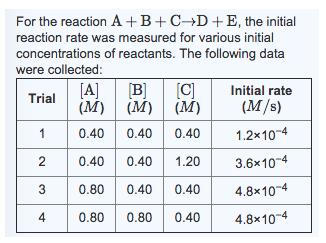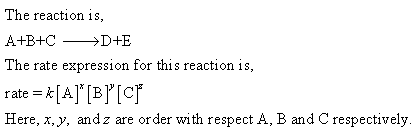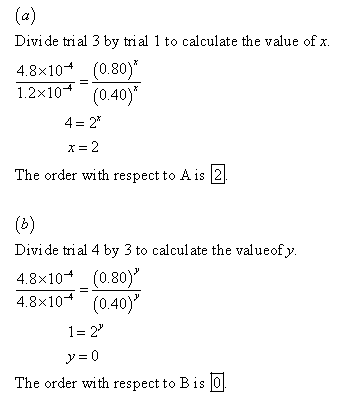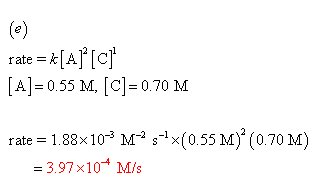# What is the reaction order with respect to A?What is the reaction order with respect to B?A. What is the reaction order with respect to A?
B. What is the reaction order with respect to B?
C. What is the reaction order with respect to C?
D. What is the value of the rate constant k for this reaction?
E. Given the data calculated in Parts A, B, C, and D, determine the initial rate for a reaction that starts with 0.55M of reagent A and 0.70M of reagents B and C?